### GCC Statement

`GCC` global_cardinality_constraint-1 <…global_cardinality_constraint-n> ;

where global_cardinality_constraint is specified in the following form:

(variables) = (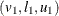<…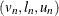> <DL= dl> <DU= du> )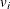is a value in the domain of one of the variables, andand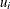are the lower and upper bounds on the number of variables assigned to. The values of dl and du are the lower and upper bounds on the number of variables assigned to values in
outside of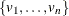.

The GCC statement specifies one or more global cardinality constraints. A global cardinality constraint (GCC) is a constraint that consists of a set of variables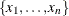and for each valuein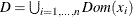, a pair of numbers
and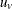. A GCC is satisfied if and only if the number of times that a valueinis assigned to the variables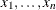is at leastand at most.

For example, the constraint that is specified with the statements

```var (x1-x6) = [1, 4];
gcc(x1-x6) = ((1, 1, 2) (2, 1, 3) (3, 1, 3) (4, 2, 3));
```

expresses that at least one but no more than two variables in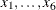can have value, at least one and no more than three variables can have value(or value), and at least two and no more than three variables can have value. For example, an assignment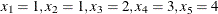, and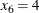satisfies the constraint.

If a global cardinality constraint has common lower or upper bounds for many of the values in, the DL= and DU= options can be used to specify the common lower and upper bounds.

For example, the previous specification could also be written as

```gcc(x1-x6) = ((1, 1, 2) (4, 2, 3) DL=1 DU=3);
```

You can also specify missing values for the lower and upper bounds. The values of dl and du are substituted as appropriate. The previous example can also be expressed as

```gcc(x1-x6) = ((1, ., 2) (4, 2, .) DL=1 DU=3);
```

The following statements specify that each of the values in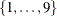can be assigned to at most one of the variables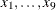:

```var (x1-x9) = [0, 9];
gcc(x1-x9) = (DL=0 DU=1);
```

Note that the preceding global cardinality constraint is equivalent to the all-different constraint that is expressed as:

```var (x1-x9) = [0, 9];
alldiff(x1-x9);
```

If you do not specify the DL= and DU= options, the default lower and upper bound for any value inthat does not appear in the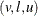format isand the number of variables in the constraint, respectively.

The global cardinality constraint also provides a convenient way to define disjoint domains for a set of variables. For example, the following syntax limits assignment of the variablesto even numbers betweenand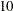:

``` var (x1-x9) = [0, 10];
gcc(x1-x9) = ((1, 0, 0) (3, 0, 0) (5, 0, 0) (7, 0, 0) (9, 0, 0));
```

If the variable list is empty, the GCC constraint applies to all the variables declared in any VARIABLE statement.